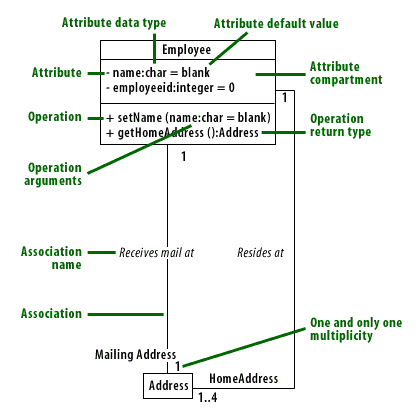Logical View  «Prev

# Elements of Class Diagrams

## Structure Diagram

As the most important example of the new structure diagram type in UML 2, the class diagram can be used by analysts, business modelers, developers, and testers throughout the software development lifecycle. This article offers a comprehensive introduction.
In UML 2 there are two basic categories of diagrams:
1. structure diagrams and
2. behavior diagrams.
Every UML diagram belongs to one these two diagram categories. The purpose of structure diagrams is to show the static structure of the system being modeled. They include the
1. class,
2. component, and
3. object diagrams.
Behavioral diagrams on the other hand, show the dynamic behavior between the objects in the system, including things like their methods, collaborations, and activities.
Example behavior diagrams are activity, use case, and sequence diagrams.

## Class Diagram in particular

UML 2 considers structure diagrams as a classification; there is no diagram itself called a Structure Diagram. However, the class diagram offers a prime example of the structure diagram type, and provides us with an initial set of notation elements that all other structure diagrams use. And because the class diagram is so foundational, we will focus on the class diagram's notation set. You should have an understanding of how to draw a UML 2 class diagram and have a solid footing for understanding other structure diagrams when we cover them later.

Here are the correct matches:1) Attribute data type, 2) Attribute default value, 3) Attribute, 4) Attribute compartment, 5) Operation, 6) Operation return type, 7) Operation arguments